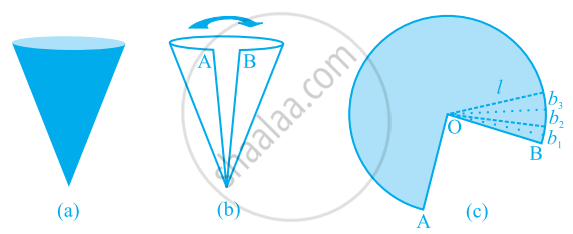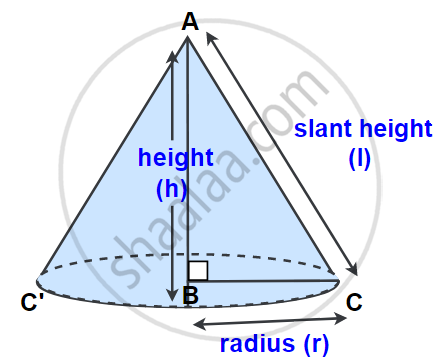# Cone:

A cone is a three-dimension solid object that has a circular base joined to point by a curved side and one vertex.## Type of Cone:

There are two types of cone:

1. Right Circular Cone:

A right circular cone is a cone whose base is a circle and whose axis is perpendicular to the base.This is called a right circular cone.

In Fig, point A is called the vertex, AB is called the height, BC is called the radius, and AC is called the slant height of the cone. Here B will be the center of the circular base of the cone. The height, radius, and slant height of the cone are usually denoted by h, r, and l respectively.

2. Oblique Cone:

A cone which has a circular base but the axis of the cone is not perpendicular with the base, is called an Oblique cone.

## Properties of Right Circular Cone:

• All elements of a right circular cone are equal.
• A section of a right circular cone that contains the vertex and two points of the base is an isosceles triangle.
• A right circular cone is a circular cone whose axis is perpendicular to its base.
• A cone has only one face, which is the circular base but no edges or vertices.
• A cone has only a vertex point.

#### description

• Cone
• Types of cone
• Properties of Right circular cone
• Terms related to a cone and their relation
If you would like to contribute notes or other learning material, please submit them using the button below.

### Shaalaa.com

Cone Definition - Altitude - Base - Slant Height - Vertex - Right Cone - Oblique Cone [00:06:47]
S
0%